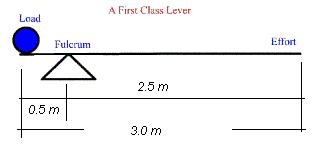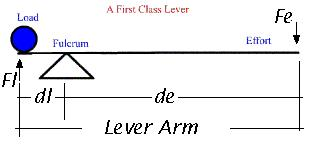#### The Law of the Lever

Archimedes of Syracuse, Italy -  was a  mathematician and physicist at the time when Sicily was a Greek colony, who lived around the year 300 BC.

One of his many inventions and discoveries was that of the lever.

The famous saying: "give me a  long enough leaver and I'll move the World", is often attributed to Archimedes.We often say in Physics that the lever is a multiplier of strength.

Levers make use of the principle of the torque where a small input of force at one end is multiplied over a long radius of action and gives a large output of force at the other end of the lever.

The simplest lever is a first class lever.

Consider an ideal first class lever.

Ideal means that we don't take into account losses due to friction, heat, sound or other energy losses; and that the total energy-work of the sytem remains constant.The law of the lever states that:

• Total Torque In = Total Torque Out
• Effort Torque = Load Torque
• Effort Force x Effort distance = Load Force x Load distance

In mathematical symbols  Fe x de = Fl x dl

Activity: Verify the law of the lever for the first class lever in the images above.